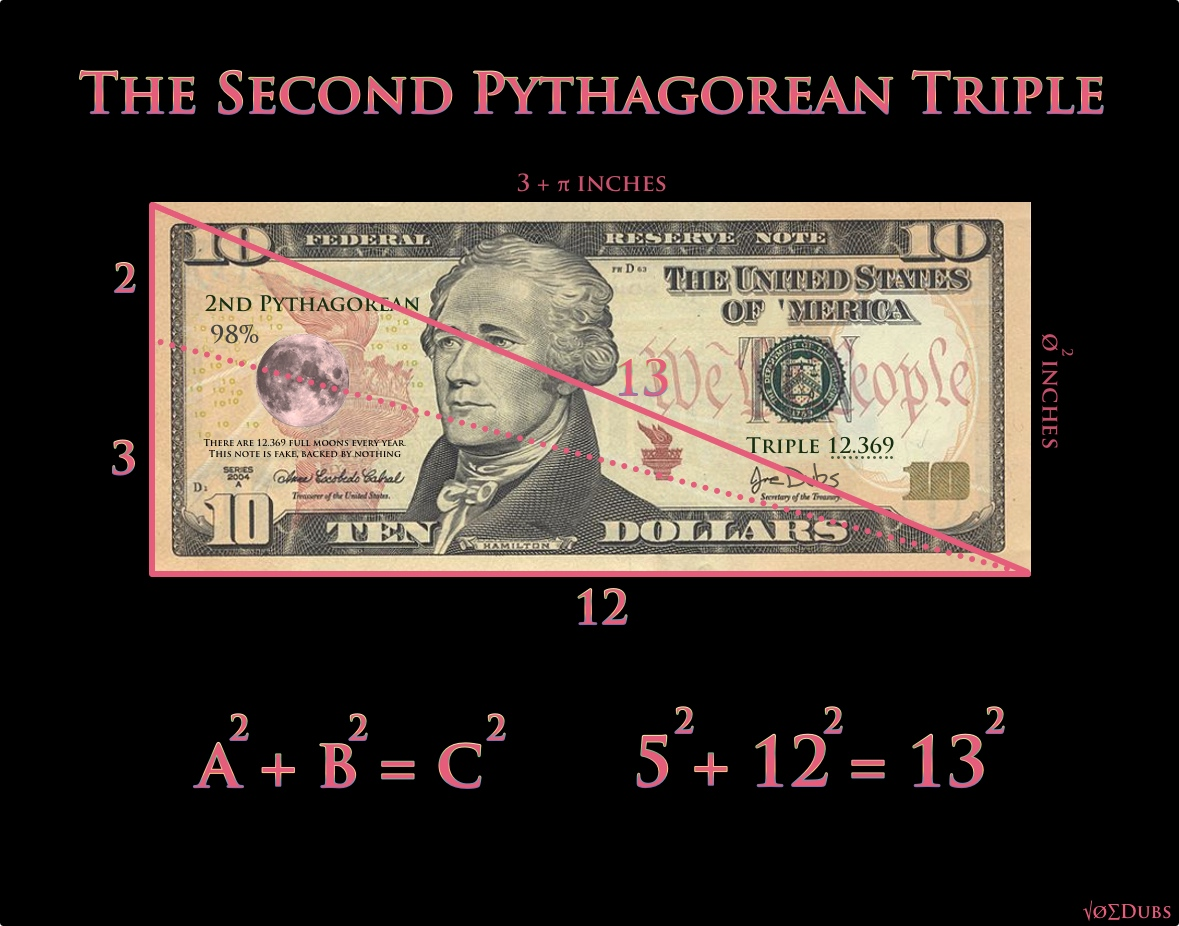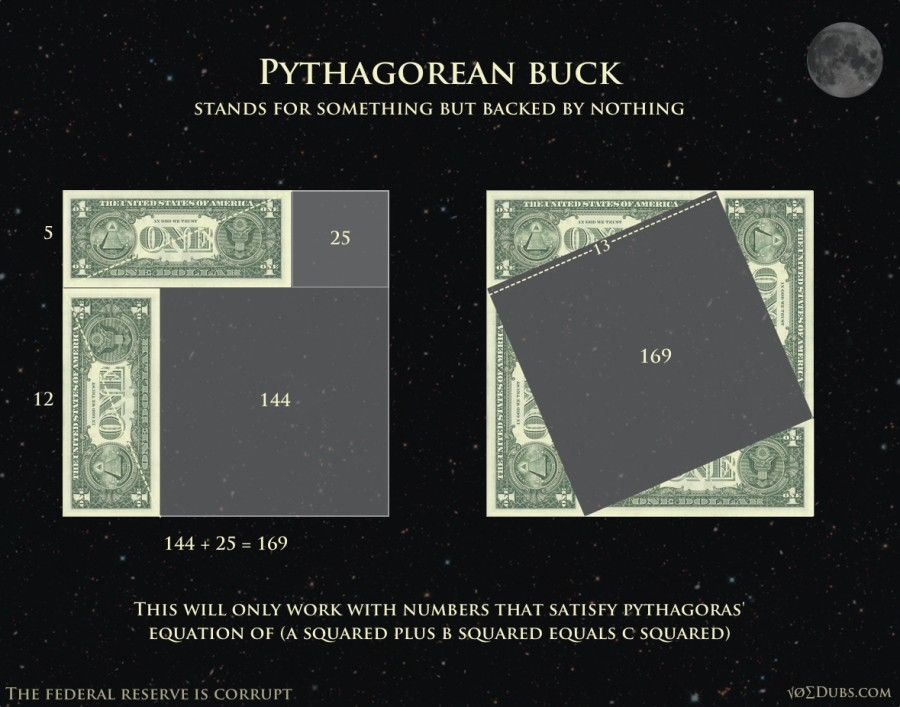# Pythagorean TriplesYou’ve heard of the Pythagorean Theorem. Looking at a triangle, A squared plus B squared equals C squared. But this doesn’t work with all triangles, only specific triangles with specific lengths. The first set of numbers that work for this formula are 3,4 and 5. (3squared=9, 4squared=16, and 5squared equals 25). 9+16=25. Check! This works, so this is the first “Pythagorean Triple”. The second triangle that satisfies the equation is based on lengths of 5,12, and 13.

Now if you divide the side of 5, into 2 and 3(The Perfect Fifth in Music Theory), and draw a line as shown(dotted line), it’s length is the amount of full moons in one solar year.(12.369)

I wonder if the privately owned Federal Reserve Bank was thinking of the Moon and the second Pythagorean Triple when they came up with the measurements of the US dollar.(The FED is not federal nor do they have any reserves.)The Moon encodes the first two Pythagorean Triples, which are sets of whole numbers that satisfy the equation popularized by Pythagoras that everyone is familiar with.

Pythagorean Theorem
a^squared + b^squared = c^squared

In the book “Taking Measure”, Scott Onstott shows that the point of intersection that defined the amount of full moons in a year (12.369), lies on the perfect fifth (2:3) ratio on the smallest side of the triangle. These numbers also encode esoteric knowledge about the geometric locations of Stonehenge and other sacred sites in England.

He continues:

“In The Lost Science of Measuring the Earth, John Michell and Robin Heath identified a 5:12:13 Pythagorean triangle that forms a greater temple around Stonehenge that I described in Secrets In Plain Sight – Volume 1. Lundy Island is the right angle point of the triangle, with Stonehenge due East, and due north the quarry in the Preseli hills of Wales where the bluestones used in Stonehenge were mined. There are 12 months in the solar year but 13 resonates with the Moon. Most years have 12 full moons but every second or third year has 13. The Moon’s dance with the Sun is complicated. Calculating the date of Easter, which is tied to both the Moon and the Sun, is a kind of calendrical rocket science. The most harmonious interval in any dance is the musical fifth. If this tone is struck it forms a just 3:2 interval. Michell and Heath drew a line from Stonehenge to the 3:2 point on the line between Lundy and Preseli and discovered that not only does it go to a giant 50 menhir on Caldey Island that a priory church is built around, but its proportion measures 12.369 which is the number of full moons in a year (99.99%). This is also the square root of the number 153 (to 5 significant digits), which has esoteric, religious, and architectural significance, see Key Numbers.”

Pythagorean Triple

Secrets in Plain SightI had to rip these dollars apart to show the significance, but don’t worry, the FED can just print more out of thin air!Our privately owned money system is completely corrupt. It is on us to come up with something better. Bitcoin is a good start!## Joe Dubs

I write about philosophy, geometry, health, politics and other stuff that interests me.